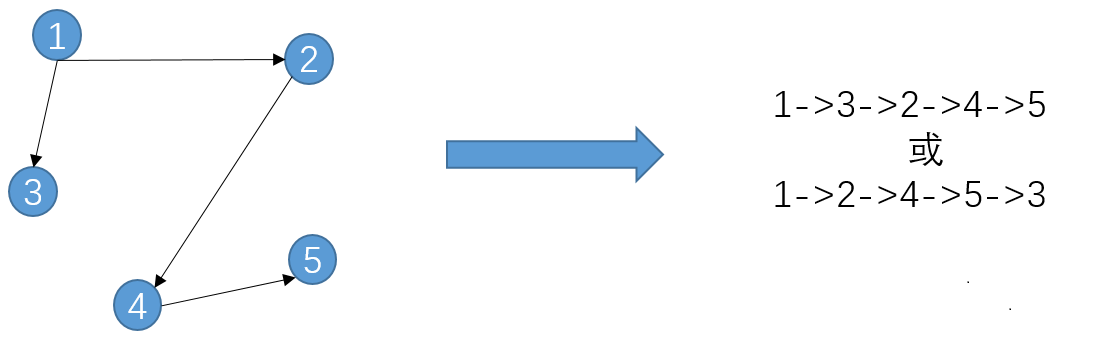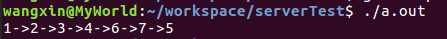• 要会写拓扑排序那得先知道什么是拓扑排序，然后是拓扑排序能干嘛，最后才是怎么实现拓扑排序。 一、什么是拓扑排序 首先拓扑排序针对的对象是一个有向无环图，将图中的节点成一个线性序列，这就是拓扑排序。线性...
今天偶然看了拓扑排序，想了一下，发现已经忘记了。于是重新炒了一下冷饭，记录一下。

要会写拓扑排序那得先知道什么是拓扑排序，然后是拓扑排序能干嘛，最后才是怎么实现拓扑排序。

一、什么是拓扑排序

首先拓扑排序针对的对象是一个有向无环图，将图中的节点排成一个线性序列，这就是拓扑排序。线性序列要求满足，图中任意一对节点若存在有向边，则必然是在的前面。满足这个条件的序列，叫拓扑序列，得到这个拓扑序列的过程叫做拓扑排序。举个栗子：可知拓扑序列并不唯一。

二、拓扑排序能干嘛

简单的说，假如要完全一个工程项目，该项目分为很多个小步骤，这些小步骤之间有先后依赖的关系，也就是说有些任务是只能先完成A，然后才能继续完成B。最终只有这些小目标都完成了，才能够达成最终的目标。这个时候，可以对这些任务，建立一个有向图，节点表示任务，有向边表示任务的先后关系。这个有向图有个官方名字，叫顶点活动图（AOV网），顶点表示活动，边表示活动时间的先后关系。

参考：百度百科-拓扑排序. https://baike.baidu.com/item/拓扑排序/5223807?fr=aladdin

举个栗子：

目标：我想用C++写一个拓扑排序。

小任务：学习C++ -> C++写程序； 了解什么是拓扑排序 -> 学习拓扑排序算法 -> 程序实现

拓扑排序（不唯一）：学习C++ --> C++写程序 -->了解什么是拓扑排序 --> 学习拓扑排序算法 --> 程序实现

构造活动的AOV网，进行拓扑排序，可确保活动可以有序进行而不会产生冲突，因为每当到达该项任务时，该任务所需的所有前驱活动已经完成。

三、拓扑排序的实现

1. 拓扑排序算法步骤

1）选择当前图中一个入度为0的顶点

2）从图中删除顶点和顶点相连的有向边

直到图中不存在入度为0的节点。

2. 拓扑排序实现

实现比较简单，这里写得比较粗糙。为了方便写，将图节点信息采用文件读取的方式进行输入，图采用邻接矩阵的方式实现，拓扑排序结果存储用vector。渐渐上头，代码如下（优化代码过程见 207. Course Schedule）：

#include <iostream>
#include <vector>
#include <fstream>
#include <cstring>
#include <cstdlib>
using namespace std;

void err(string str)
{
cout <<"error: " <<str <<endl;
exit(-1);
}

void topo(int **graph, int *degree, vector<int>& res)
{
int len = res.size();
int node=0, index = 0;
while(node <= len){
for(node=1;node<=len;node++){
//每次寻找一个入度为0的节点
if(degree[node] == 0) {
degree[node] = -1;
res[index++] = node;    //存入vector
break;
}
}
if(node <= len){    // 删除与节点相关的边，同时将目的节点的入度减少
for(int i=1;i<=len;i++){
if(graph[node][i] == 1){
degree[i]--;
graph[node][i] = 0;
}
}
}
}
}

int main()
{
// 图节点设置由文件读入
fstream ff("node.txt");
if(!ff.is_open()){
err("open error");
}
int nodeNums = 0;
ff >>nodeNums;        //    获取节点数

// 只是突然想写一下二维数组的动态分配
/**
*    图用邻接矩阵的方式存储，其实稀疏图用链表存储更好
**/
int **graph;
graph = (int **)malloc(sizeof(int*)*(nodeNums+1));
for(int i=0;i<=nodeNums;i++)
graph[i] = (int*)malloc(sizeof(int)*(nodeNums+1));

int degree[nodeNums+1] = {0};    //存储每个节点入度
int n1, n2;
while(!ff.eof()){
ff >>n1 >>n2;
if(n1 > nodeNums || n2 > nodeNums) err("node error");
graph[n1][n2] = 1;       //图的初始化
degree[n2]++;
}
// for(int i=0;i<=nodeNums;i++)
//      cout <<degree[i] <<endl;
// cout <<endl;

vector<int> res(nodeNums);    //vector用于存储最终的拓扑节点，预先分配vector大小
topo(graph, degree, res);

for(int i=0;i<nodeNums-1;i++){
cout <<res[i] <<"->";
}
cout <<res[nodeNums-1] <<endl;
return 0;
}


图配置如下：

7
1 3
1 4
3 6
3 7
4 7
7 5

实现结果：展开全文• 以前不知道什么是拓扑排序，最近补充了一下知识才算是知道怎么回事。 概念如下：对一个有向无环图(Directed Acyclic Graph简称DAG)G进行拓扑排序，是将G中所有顶点成一个线性序列，使得图中任意一对顶点u和v，若边...
以前不知道什么是拓扑排序，最近补充了一下知识才算是知道怎么回事。
概念如下：对一个有向无环图(Directed Acyclic Graph简称DAG)G进行拓扑排序，是将G中所有顶点排成一个线性序列，使得图中任意一对顶点u和v，若边(u,v)∈E(G)，则u在线性序列中出现在v之前。通常，这样的线性序列称为满足拓扑次序(Topological Order)的序列，简称拓扑序列。简单的说，由某个集合上的一个偏序得到该集合上的一个全序，这个操作称之为拓扑排序。
说起来比较复杂，其实应用很为广泛。比如生活中的一些事情都是拓扑排序的结果。
比如，早上吃饭前需要刷牙，洗脸，等等就可以表示成下图。
刷牙->吃饭
洗脸->吃饭
吃饭->上班
穿衣->上班
排序的结果就是 刷牙->洗脸->吃饭->穿衣->上班，这么一个过程。拓扑排序可以形象的表示各个子过程之间的关系。有了这个例子觉得编译器中依赖解析什么的都先对这些东西判断是否存在环吧。

package q1175;

import java.util.Scanner;
import java.util.Set;
import java.util.HashMap;
import java.util.HashSet;
import java.util.Map;
import java.util.Iterator;

/**
* Author: Ethan(Baiyp) <br />
* Date: 2015/7/9. <br />
*/
public class Main {
public static void main(String[] args) {
Scanner in = new Scanner(System.in);
while (in.hasNext()) {
String input;
input = in.next();

int n = Integer.parseInt(input);
input = in.next();
int m = Integer.parseInt(input);
input = in.next();
int k = Integer.parseInt(input);
input = in.next();
int initValue = Integer.parseInt(input);

Graph g = new Graph(n);

g.init(k, initValue);

while (m-- > 0) {
input = in.next();
int start = Integer.parseInt(input);
input = in.next();
int end = Integer.parseInt(input);
g.insertEdge(new Edge().setStart(start).setEnd(end));
}
g.topoSort();
System.out.println(g.getTotal());
}
}

private static class Graph {
private Map<Integer, Set<Edge>> edgeMap;
private Map<Integer, Vertex> vertexMap;
private int total;

public Graph(int num) {
total = 0;
edgeMap = new HashMap<Integer, Set<Edge>>();
vertexMap = new HashMap<Integer, Vertex>();
for (int i = 1; i <= num; i++) {
edgeMap.put(i, new HashSet<Edge>());
vertexMap.put(i, new Vertex().setIndex(i).setWeight(0));
}
}

public void insertEdge(Edge edge) {
int index = edge.getEnd().getIndex();
edgeMap.get(index).add(edge);
}

public void init(int key, int value) {
vertexMap.get(key).setWeight(value);
}

public void topoSort() {
for (int i = 0; i < vertexMap.size(); i++) {
int temp = vertexMap.get(i + 1).getWeight();
Set<Edge> set = edgeMap.get(i + 1);
Iterator<Edge> iterator = set.iterator();

while (iterator.hasNext()) {
Edge e = iterator.next();
temp += vertexMap.get(e.getStart().getIndex()).getWeight();
}

init(i + 1, temp);
total += temp;
}
}

public int getTotal() {
return total;
}
}

/**
* Directed Edge
*/
private static class Edge {
private Vertex start;
private Vertex end;

public Edge setStart(int start) {
this.start = new Vertex().setIndex(start).setWeight(0);
return this;
}

public Edge setEnd(int end) {
this.end = new Vertex().setIndex(end).setWeight(0);
return this;
}

public Vertex getStart() {
return start;
}

public Vertex getEnd() {
return end;
}
}

private static class Vertex {
private int index;
private int weight;

public int getIndex() {
return index;
}

public Vertex setIndex(int index) {
this.index = index;
return this;
}

public int getWeight() {
return weight;
}

public Vertex setWeight(int weight) {
this.weight = weight;
return this;
}
}
}
其中的Edge为一条边，为有向边，整个图也是通过对边的映射，对顶点的映射来完成。其中顶点中包含的weight就是拓扑排序中的权重，只需要在写一个compareTo接口，就可以使用泛型针对其进行排序了。

图中对边的索引为通过结束顶点来对边进行索引，因为有向边的结束顶点的权重为其起始顶点的权重之和。

展开全文JAVA 算法
• ## 拓扑排序dfs版+判环

千次阅读 2018-09-15 13:30:00
以前就听说拓扑排序可以用dfs来写了，只是一直没有去尝试，想一想的话会觉得很复杂，dfs怎么排？ 要从入度为0的点出发吗？ 如果有多个入度为0的点，每个都dfs一遍吗？那他们不是会有重复不是会乱套？ 总之，对于...
以前就听说拓扑排序可以用dfs来写了，只是一直没有去尝试，想一想的话会觉得很复杂，dfs怎么排？

要从入度为0的点出发吗？
如果有多个入度为0的点，每个都dfs一遍吗？那他们不是会有重复不是会乱套？

总之，对于从来都是用bfs写拓扑的我来说，觉得用dfs简直不可思议。但是了解之后，买毛病！精彩！而且还学会了一张图如何判环（因为有环的图是不能拓扑排序的）。

总体思路就是：dfs + 栈。

首先我们讨论一下拓扑排序的性质，对于一个图，它可能会有好几种拓扑排序，但他们同时满足一个规律，那就是如果存在有向边u->v, 那么结点u必须排在v之前（前驱）。同时这种性质具有传递性，也就是说如果同时存在v->t, 那么满足u在t之前。同样的，如果u和v两个结点在图中并不满足这种性质，那么谁在前谁在后就无所谓了。正是利用这个规则，我们进行dfs的顺序是无所谓的。

为何？因为我们从root结点开始dfs一遍，可以找到所有的必须在这个root结点之后的点，那么我们就满足了拓扑序的规则了，那么我们无论先dfs(u)还是先dfs(v), 都不会违背这个规则（除非有环），那么同时我们只要按照某种合理的方式存储所有这些点，那么他们就是拓扑序了。

什么是合理的方式？栈！考量一个dfs(u), 在它结束该退出时，它代表它的结点u。在dfs递归中，什么点会最先exit？没有后继结点的点（或者后继已入栈的点）！那么把所有点分成两个集合，一个是待处理的点集D，一个是已拓扑排序后的点集A，当且仅当D中某个点没有后继结点（或该后继结点已经加入了点集A中）时，它可以从D转移到A，而dfs的回溯方式，恰恰就自动实现了这样的功能。 结合代码更容易体会。

不判环拓扑排序代码，如果你已知图是DAG的话。

#include <iostream>
#include <stack>
using namespace std;

struct Edge {
int to, next;
};

const int maxn = 10010;
int head[maxn] = {};
int n, m, cnt = 1;
bool vis[maxn] = {};
Edge edge[maxn];
stack<int> S;

void add(int u, int v)
{
edge[cnt].to = v;
edge[cnt].next = head[u];
head[u] = cnt++;
}

void dfs(int u)
{
vis[u] = true;
for (int i = head[u]; i; i = edge[i].next) {
int v = edge[i].to;
if (!vis[v]) dfs(v);
}
S.push(u);
}

int main()
{
cin >> n >> m;
for (int i = 1; i <= m; ++i) {
int u, v;
cin >> u >> v;
add(u, v);
}
for (int i = 1; i <= n; ++i) {
if (vis[i] == 0) dfs(i);
}
while (!S.empty()) {
cout << S.top() << ' ';
S.pop();
}
}

那么，如何判环呢？判环只是在dfs函数上稍做些修改，其中最主要的是vis数组的含义有所扩展，以及对下一结点进行dfs的条件判断。

不判环的拓扑排序，vis只代表某一结点有没有被放问过，而现在，vis有三个值，-1，0，1。-1代表已访问过，但不是从当前系列dfs访问来的，0代表未访问过，1代表访问国，且是当前系列访问过的（意味着有环，如u->v, v->t, t->u）

核心代码如下

bool dfs(int u)
{
vis[u] = 1;
for (int i = head[u]; i; i = edge[i].next) {
int v = edge[i].to;
if (vis[v] == 1) return false;
if (vis[v] == 0 && !dfs(v)) return false;
}
vis[u] = -1;
S.push(u);
return true;
}

dfs（）函数的值意味着是否有环。

可以看到，如果发现将要访问的节点在这个dfs圈子里（为1），那么直接返回false，否则如果没访问过（为0），那么就进去访问吧，不过因为我们要维护是否有环的性质，所以对其返回值进行判断。如果为false，对不起，有环，拓扑排序直接终止了。

完整代码：

#include <iostream>
#include <stack>
using namespace std;

struct Edge {
int to, next;
};

const int maxn = 10010;
int n, m, cnt = 1;
int head[maxn] = {};
int vis[maxn] = {};
Edge edge[maxn];
stack<int> S;

void add(int u, int v)
{
edge[cnt].to = v;
edge[cnt].next = head[u];
head[u] = cnt++;
}

bool dfs(int u)
{
vis[u] = 1;
for (int i = head[u]; i; i = edge[i].next) {
int v = edge[i].to;
if (vis[v] == 1) return false;
if (vis[v] == 0 && !dfs(v)) return false;
}
vis[u] = -1;
S.push(u);
return true;
}

bool topsort()
{
for (int i = 1; i <= n; ++i) {
if (!vis[i]) if (!dfs(i)) {
return false;
}
}
return true;
}

int main()
{
cin >> n >> m;
for (int i = 1; i <= m; ++i) {
int u, v;
cin >> u >> v;
add(u, v);
}
bool ans = topsort();
if (ans == false) cout << "图中存在环，不能进行拓扑排序" << endl;
else {
while (!S.empty()) {
cout << S.top() << ' ';
S.pop();
}
}
}
展开全文• 坑的地方就是刚好只学了dfs怎么写于是折腾了很久也不知道怎么办，最后花了五分钟学了一下bfs的拓扑排然后五分钟过了两题= = 两题的注释AC代码如下 class Solution { public: bool canFinish(in...
课程表①和课程表②两题几乎一模一样

思想不多说都给好了纯粹的模板题，比较恶心的是用dfs拓扑排判断是否存在有向环非常麻烦（不麻烦的也有但太菜不会

坑的地方就是刚好只学了dfs怎么写于是折腾了很久也不知道怎么办，最后花了五分钟学了一下bfs的拓扑排然后五分钟过了两题= =

两题的注释AC代码如下

class Solution
{
public:
bool canFinish(int numCourses, vector<pair<int, int>>& prerequisites)
{
//度数，即有多少个点有边指向它，或者说它的前驱有多少个点
vector<int> degree(numCourses,0);

//建图，邻接表，相当于vector<edge> G[MAX_N]
vector<vector<int>> G(numCourses, vector<int>());
for(auto &p:prerequisites)
{
G[p.second].push_back(p.first);
degree[p.first]++;
}
int n=numCourses;
queue<int> q;
//度数为0的，即没有前驱的，当然就是拓扑序最前的
for(int i=0;i<numCourses;i++)
if(degree[i]==0)
q.push(i);
while(!q.empty())
{
int cur=q.front();
q.pop();
//表示最前面的一个点已经安排上了，n--
n--;
//cur已经出队了，G[cur]中的所有点，即cur的所有后继的度数应该减1
//顺便判断一下是否为0，是就入队了，否则还要再循环一遍麻烦
for(auto &next:G[cur])
if(--degree[next]==0)
q.push(next);
}
//如果为无环有向图最后肯定循环到n为0
//有环就反之，中间一步导致没有元素可入队，n！=0
return n==0;
}
};

第二题同上，就是多了个vector保存拓扑排序后的序列，每次在尾部插入，正好就是拓扑序。注意如果此处是用dfs，那就需要每次在头部插入了，因为dfs的思想是先找无后继的，然后向前回溯，而bfs是先找无前驱的，然后向后依次处理。

class Solution
{
public:
vector<int> findOrder(int numCourses, vector<pair<int, int>>& prerequisites)
{
vector<int> degree(numCourses,0);
vector<vector<int>> G(numCourses, vector<int>());
for(auto &p:prerequisites)
{
G[p.second].push_back(p.first);
degree[p.first]++;
}
vector<int> ans;
int n=numCourses;
queue<int> q;
for(int i=0;i<numCourses;i++)
if(degree[i]==0)
q.push(i);
while(!q.empty())
{
int cur=q.front();
ans.push_back(cur);
q.pop();
n--;
for(auto &next:G[cur])
if(--degree[next]==0)
q.push(next);
}
if(n==0)
return ans;
else
return {};
}
};


展开全文• 这题挺奇怪的，我之前还真么做过这样的拓扑排序，之前卡了一阵子，这个题目是判断到第几个成环，到第几个正好能唯一排序，另一种就是怎么不了序，就是每个数据跑一遍拓扑排序就行了#include #include #include...
• 火星人的血缘关系很奇怪，一个人可以有很多父亲，当然一个人也可以有很多孩子。...（大概我的智商真的不合适，否则怎么这么久了连个拓扑排序都写不好，T了三次。。） 代码： /****************...
• 马上要上体育课了，上体育课之前总归是要个队的，ly作为班长，怎么排队的问题只能由她来解决，但是马上要上课了，ly又不清楚所有人的身高，她又不好意思问每个人的身高，因为这样会显的自己很不负责，于是她只能...
• 题意： ...//我们考虑一下怎么排好一个位置，这个位置可能有多个东西可以占，那么这些东西对于这个位置都是等价的， //那么我们可以采用深搜下+回溯来解决。 //其实还是蛮简单的 code： #inclu...
• 显然模拟拓扑排序的步骤是必不可少了。 假设我们当前有t个点，他们的入度均为0.我们不知道该选取哪一个。 我们把这t个点按从小到大好序（放入小顶堆），假设我们目前有k条边（k 现在问题来了，我们给前k个点加一...
• 2153: D.ly的排队问题Time Limit: 1 Sec Memory Limit: 128 MBSubmit: 35 Solved: 13[Submit][Status][Web Board]Description马上要上体育课了，上体育课之前总归是要个队的，ly作为班长，怎么排队的问题...
• 马上要上体育课了，上体育课之前总归是要个队的，ly作为班长，怎么排队的问题只能由她来解决，但是马上要上课了，ly又不清楚所有人的身高，她又不好意思问每个人的身高，因为这样会显的自己很不负责，于是她只能...
• 【题目】 2153: D.ly的排队问题 Time Limit: 1 SecMemory ...马上要上体育课了，上体育课之前总归是要个队的，ly作为班长，怎么排队的问题只能由她来解决，但是马上要上课了，ly又不清楚所有人的身高，她又不好...优先队列
• 马上要上体育课了，上体育课之前总归是要个队的，ly作为班长，怎么排队的问题只能由她来解决，但是马上要上课了，ly又不清楚所有人的身高，她又不好意思问每个人的身高，因为这样会显的自己很不负责，于是她只能...
• 马上要上体育课了，上体育课之前总归是要个队的，ly作为班长，怎么排队的问题只能由她来解决，但是马上要上课了，ly又不清楚所有人的身高，她又不好意思问每个人的身高，因为这样会显的自己很不负责，于是她只能...优先队列 字典序最小
• 拓扑排序后，可以发现，删除层数靠后的点会对前面产生影响，因为此时想统计前面的边存在的最长路就不能判掉经过这个点的路径，所以只能按拓扑序从前往后删点。 这里直接说做法吧，维护一个大根堆，储存当前枚...
• 好了，不扯了，看看度娘怎么讲的吧：对一个有向无环图(Directed Acyclic Graph简称DAG)G进行拓扑排序，是将G中所有顶点成一个线性序列，使得图中任意一对顶点u和v，若 ∈E(G)，则u在线性序列中出现在v之前。...
• 　过了两天，不管怎么说参考着一些别人的程序总算是写完了，大循环以位置为顺序一个个试人试下去，SCDP小循环以状态为顺序并考虑拓扑排序（这时候是以人的顺序来位置的）实现状态转移。很长的一句话其实就是对于每...
• 你上了公交车发现前有两个空座位，而后排所有座位都已经坐满，你会怎么做？立马下车，并对自己说，后面没座了，我等下一辆？没这么笨的人，前面有座位，当然也是可以坐的。 4.12.1队列顺序存储的不足 112 4.12.2...数据结构